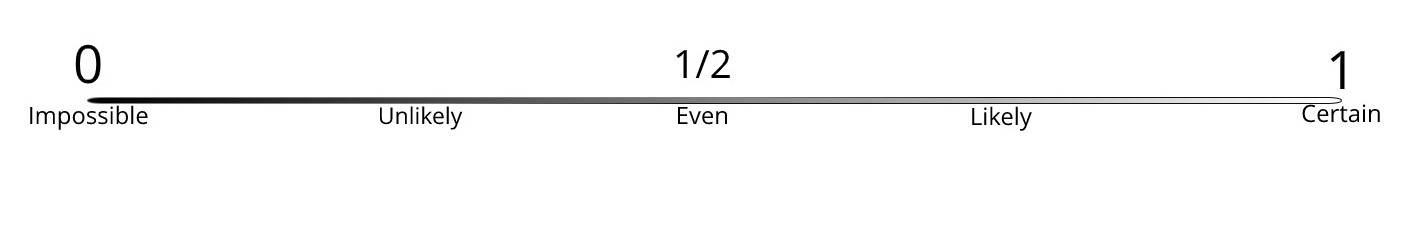# 1.4. Probability Scale

Last updated: May 26th, 2020
In :
import pandas as pd


# Probability Scale¶

Let's use one more time our small dataset, and our random experiment of picking a person and printing their city:

In :
dataset =  pd.DataFrame({
'Person #':[1,2,3,4,5,6,7,8,9,10],
'City':['SF','SF','NY','NY','NY','SF','NY','SF','SF','SF'],
'Age':[41,26,28,53,32,51,65,49,25,33]
})
dataset

Out:
Person # City Age
0 1 SF 41
1 2 SF 26
2 3 NY 28
3 4 NY 53
4 5 NY 32
5 6 SF 51
6 7 NY 65
7 8 SF 49
8 9 SF 25
9 10 SF 33
In :
dataset.sample(1)['City'].values

Out:
'SF'

We have said that the probability of an outcome is the likelihood of getting that outcome. Now what if we are certain that a given outcome cannot happen?

For example, what would be the probability of the person chosen being from Washington?

Since the likelihood is null, the probability of an impossible outcome will be $0$.

This can be seen in terms of relative frequency as well, because if the outcome is impossible, the proportion of times we will get that result is $0$.

And we want the probability to increase as the likelihood of the outcome increases, until we reach the other end where we are certain that our outcome will happen.

For example, what would be the probability of the person chosen being from USA?

Again, if we think about it as a relative frequency, we are calculating the amount of successful experiments over the total amount of experiments performed. But in this case they are equal, so that proportion is $1$.

This means that the probability of a certain outcome will be $1$.

This leads us to our first important result:

### For any $\omega\in\Omega$, $0\leq P(\omega)\leq 1$.¶

In words this means that the probability of any outcome will be a number between $0$ and $1$.

It can be given as a fraction (e.g. $1/3$), or as a decimal (e.g. $0.7$), but it can never be negative and it can never be larger than $1$.

With this, we can think of probability as a scale of likelihood:One final remark: sometimes we think of probabilities in terms of percentages, for example the probability of rain tomorrow is of $40\%$, but that only means $40$ times out of a hundred, so we can write it as $\frac{40}{100}$ which is $0.4$.